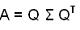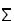## Fast SVD of Hankel/Toeplitz and Takagi Factorization Package (MatLab)

### 1. Introduction

For any symmetric matrix A, there exists a special form of SVD, called Takagi factorization:where Q is unitary andis the diagonal singular value matrix.

This package of Matlab functions computes the Takagi factorization of a complex-symmetric matrix. The computation consists of two stages: Lanczos tridiagonalization and symmetric SVD of a symmetric and tridiagonal matrix. Three Lanczos tridiagonalization functions are provided. The first uses partial orthogonalization, and the second uses modified partial orthogonalization. The third uses modified partial orthogonalization with an added restart technique. In general, the modified partial orthogonalization versions are more efficient than the partial orthogonalization version. The modified partial orthoganlization with the restart technique is comparatively more robust. Three methods for symmetric SVD of a symmetric and tridiagonal matrix are provided. One uses pure implicit QR method, another uses the divide-and-conquer method and another uses the twisted factorization method. Usually, the twisted factorization method is much faster than the divide-and-conquer method which is in turn usually much faster than the implicit QR method. The implicit QR method uses the order two class two shift, since we implicitly solve a symmetric pentadiagonal eigenvalue problem.

The matrix-vector multiplication in the Lanczos functions can be replaced by a matrix-vector multiplication function. For example, if it is replaced by a fast Hankel matrix-vector multiplication, this package can be used for fast Hankel SVD. If it is replaced by a sparse symmetric matrix-vector multiplication, this package can be used for sparse symmetric SVD. Since a Toeplitz matrix can be transformed into a Hankel by reversing its columns or rows, this package can also be used for fast Toeplitz SVD.

### 2. Dependency

```                                     TakagiDemo
_________ _________________________|____________________________________________________________________________________
|         |                         |                                                |                                   |
csgen     LanMPOR                  cstsvdd (divide-and-conquer)                     cstsvdt (twisted)                    CSSVD (QR)
|       LanMPO            __________|__________                      ________________|______________                     |
unitarand   LanPO            |                     |                    |          |          |         |                 cssvdstep
sqevd                eig2tak              cssing      cstlqd     twist    eig2tak          _______|_______
______|_______                                   |          |          |                      |       |       |
|              |                             cssingstep    rotate      cqds                  cssvd2  house   shift22
CSSVD          sevr                            _____|_____                                       |               |
______|______                      |           |                                    csvd2           rotate
|             |                   cssvd2      shift22                             _____|_____
deflate         slv                    |           |                                |           |
|                                 csvd2       rotate                          rotate      slasv2
rotate                             ____|____
|         |
rotate    slasv2
```
` `
```

FHSVDDemo
_________________________|___________________________________________________________________________________
|                         |                                                |                                  |
FHLanMPOR                 cstsvdd (divide-and-conquer)                     cstsvdt (twisted)                   CSSVD (QR)
FHLanMPO           __________|__________                      ________________|______________                    |
|              |                     |                    |          |          |         |                cssvdstep
fhmvmul         sqevd                eig2tak              cssing      cstlqd     twist    eig2tak         _______|_______
______|_______                                   |          |          |                     |       |       |
|              |                             cssingstep    rotate      cqds                 cssvd2  house   shift22
CSSVD          sevr                            _____|_____                                      |               |
______|______                      |           |                                   csvd2           rotate
|             |                   cssvd2      shift22                            _____|_____
deflate         slv                    |           |                               |           |
|                                 csvd2       rotate                         rotate      slasv2
rotate                             ____|____
|         |
rotate    slasv2

```

### 3. Functions

 cqds.m Compute complex quotient-differences with shift, given Cholesky factorization. csgen.m Generate a random complex-symmetric matrix, given its singular values. cssing.m The singular values of a complex symmetric tridiagonal T given by its diagonals. cssingstep.m One QR step in complex symmetric SVD, computes singular values only. CSSVD.m The SVD of a tridiagonal complex-symmetric matrix using implicit QR method with order two class two shifts. cssvd2.m Compute the SVD of a 2-by-2 complex-symmetric matrix. cssvdstep.m One step of the implicit QR method for tridiagonal complex-symmetric matrices using order two class two shift. cstlqd.m Compute the LQ decomposition of a complex-symmetric and tridiagonal matrix. cstsvdd.m The symmetric SVD of a symmetric and tridiagonal matrix using divide-and-conquer method. cstsvdt.m The symmetric SVD of a symmetric and tridiagonal matrix using twisted factorization method. csvd2.m The SVD of a 2-by-2 complex matrix. deflate.m Deflation in the symmetric eigenvalue rank-one modification problem. eig2tak.m Convert eigenvalues and eigenvectors (left singular vectors) into Takagi values and Takagi vectors. FHLanMPO.m Fast Lanczos tridiagonalization of Hankel matrices using the modified partial orthogonalization. FHLanMPOR.m Fast Lanczos tridiagonalization of Hankel matrices using the modified partial orthogonalization and restart. fhmvmul.m Fast Hankel matrix-vector multiplication. FHSVDDemo.m Demonstrate the fast Hankel SVD. house.m Householder transformation given a vector. LanMPO.m Lanczos tridiagonalization of a complex-symmetric matrix using the modified partial orthogonalization. LanMPOR.m Lanczos tridiagonalization of a complex-symmetric matrix using the modified partial orthogonalization and restart. LanPO.m Lanczos tridiagonalization of a complex-symmetric matrix using the partial orthogonalization. rotate.m Rotation transformation given a 2-D vector. sevr.m Symmetric eigen problem rank-one modification. shift22.m Find the order two class two shift. slasv2.m Compute the SVD of a 2-by-2 real and upper triangular matrix. slv.m Secular equation solver. sqevd.m Symmetric pentadiagonal eigenvalue decomposition. The symmetric pentadiagonal matrix is a product of a tridiagonal matrix and its transpose. TakagiDemo.m Demonstrate the Takagi factorization, or symmetric SVD, of a complex-symmetric matrix. twist.m Compute the corresponding eigenvector using twisted factorization. unitarand.m Generate a random unitary matrix.

### 4. References

• Wei Xu and Sanzheng Qiao, A Twisted Factorization Method for Symmetric SVD of a Complex Symmetric Tridiagonal Matrix, Technical Report No. CAS 06-01-SQ, Department of Computing and Software, McMaster University, Hamilton, Ontario, Canada. L8S 4K1. January 2006. ( ps, pdf )
• Wei Xu and Sanzheng Qiao, A Divide-and-Conquer Method for the Takagi Factorization, Technical Report No. CAS 05-01-SQ , Department of Computing and Software, McMaster University, Hamilton, Ontario, Canada. L8S 4K1. February 2005. ( ps, pdf )
• Chengshu Guo and Sanzheng Qiao, A Stable Lanczos Tridiagonalization of Complex Symmetric Matrices,Technical Report No. CAS 03-08-SQ , Department of Computing and Software, McMaster University, Hamilton, Ontario L8S 4K1, Canada. July 2003. (Revised, September 2003.) ( ps, pdf)
• F.T. Luk and S. Qiao, A fast singular value algorithm for Hankel matrices, Fast Algorithms for Structured Matrices: Theory and Applications, Contemporary Mathematics 323, Editor V. Olshevsky, American Mathematical Society. 2003. 169--177. ( ps, pdf)